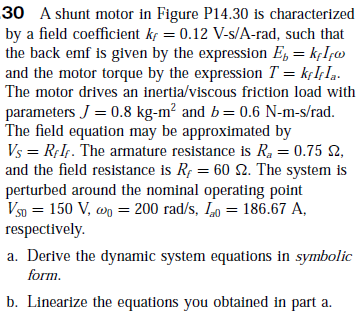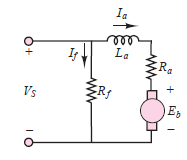# 30 A shunt motor in Figure P14.30 is characterized by a field coefficient kf = 0.12 V-s/A-rad, such that the back emf is given by the expression E, = kfIfw and the motor torque by the expression T = kçI,Iq. The motor drives an inertia/viscous friction load with parameters J = 0.8 kg-m² and b= 0.6 N-m-s/rad. The field equation may be approximated by Vs = R;IF. The armature resistance is R, = 0.75 2, and the field resistance is Rp = 60 2. The system is perturbed around the nominal operating point V5p = 150 V, wn = 200 rad/s, Io = 186.67 A, respectively. %3D a. Derive the dynamic system equations in symbolic form. b. Linearize the equations you obtained in part a. Ia ll La Ra Vs ER

Questionhelp_outlineImage Transcriptionclose30 A shunt motor in Figure P14.30 is characterized by a field coefficient kf = 0.12 V-s/A-rad, such that the back emf is given by the expression E, = kfIfw and the motor torque by the expression T = kçI,Iq. The motor drives an inertia/viscous friction load with parameters J = 0.8 kg-m² and b= 0.6 N-m-s/rad. The field equation may be approximated by Vs = R;IF. The armature resistance is R, = 0.75 2, and the field resistance is Rp = 60 2. The system is perturbed around the nominal operating point V5p = 150 V, wn = 200 rad/s, Io = 186.67 A, respectively. %3D a. Derive the dynamic system equations in symbolic form. b. Linearize the equations you obtained in part a. fullscreenhelp_outlineImage TranscriptioncloseIa ll La Ra Vs ER fullscreen

### Want to see this answer and more?

Experts are waiting 24/7 to provide step-by-step solutions in as fast as 30 minutes!*

*Response times may vary by subject and question complexity. Median response time is 34 minutes for paid subscribers and may be longer for promotional offers.
Tagged in
Engineering
Electrical Engineering

### Control Systems